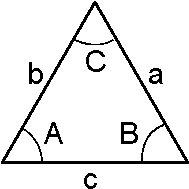# Law of Cosines Formula

If the two sides and angles of the triangle are given, then the unknown side and angles can be calculated using the cosine law. Law of cosine is another formula used to find out the unknown side of the triangle.The Law of Cosine Formula is,

$\large a^2=b^2+c^2-2(bc)Cos\;A$ $\large b^2=a^2+c^2-2(ac)Cos\;B$ $\large c^2=a^2+b^2-2(ab)Cos\;C$The cosine law can be derived out of Pythagoras Theorem.

The Pythagorean theorem can be derived from the cosine law. In the case of a right triangle the angle, θ = 90°. So, the value of cos θ becomes 0 and thus the law of cosines reduces to

$$\begin{array}{l}c^2=a^2+b^2\end{array}$$

## Law of Cosines Problem

Some solved problem on the law of cosines are given below:

### Solved Examples

Question 1: Given the sides of the triangle b = 7 cm; c = 8 cm and the angle A = 45o. Calculate the unknown sides and angles ?
Solution:

Given,
b = 7 cm
c = 8 cm
A = 45o
The law of cosines formula is: a2 = b2 + c2 – 2bc cos A
a2 = (7 cm)2 + (8 cm)2 – 2(7 cm)(8 cm) cos 45
a2 = 49 cm2 + 64 cm2 – (112 cmx 0.707)
a2 = 49 cm2 + 64 cm2 – 79.18 cm2
a2 = 33.82
a = 5.82 cm
b2= a2 + c2 – 2ac cos B72 = (5.82)2 + 82 – 2(5.82)(8) cos B

49 = 33.8724 + 64 – 93.12 cos B

93.12 cos B = 48.8724

Cos B = 48.8724/93.12

B = 58.3o

c2 = a2+ b2 – 2ab cos C

82 = (5.82)2 + 72 -2(5.82)(7) cos C

64 = 33.8724 + 49 – 81.48 cos C

81.48 cos C = 18.8724

Cos C = 18.8724/81.48

C = 76.6o